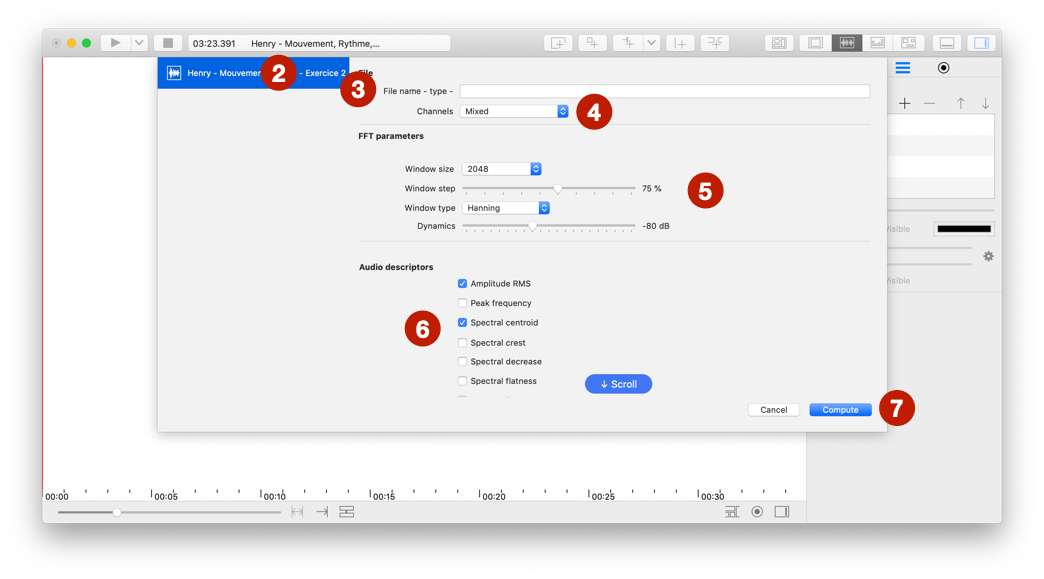# Audio descriptors

## Compute descriptors

Before use this feature, you need to import audio or movie file.1. Select `Compute` > `Audio Descriptors`.
2. Select a file.
3. [Optional] Edit title of file.
4. Mix channels before computing or compute descriptors on each channel in different series.
5. Change `FFT parameters`.
6. Select one or more descriptors and edit parameters.
7. Select `Compute`.

You can use descriptor files in temporal visualization or timeline

## Types of descriptors

### Amplitude RMS

Compute root mean square amplitude of signal (No FFT parameters is needed).

### Fundamental frequency estimation

Compute f0 for each frame. This command uses YIN algorithm and is adapted to solo voice or monophonic instrument.

### Peak frequency

Compute peak of frequency for each frame.

### Spectral centroid

Compute the centre of gravity of FFT.

### Spectral crest

Ratio between the maximum value and the arithmetic mean of the FFT.

### Spectral decrease

Average spectral slope of the FFT.

### Spectral flatness

Ratio between the geometric mean and the arithmetic mean.

### Spectral flux

Temporal variation of the logarithmically-scaled rate-map across adjacent frames.

### Spectral rolloff

Frequency in below which the threshold of the total spectral energy is concentrated.

### Spectral standard deviation

Square root of the spectral variance.

### Spectral variance

Measures how much the frequencies can deviate from the centre of gravity (spectral centroid).

### Zero crossing rate

Rate at which zero crossings occur (No FFT parameters is needed).